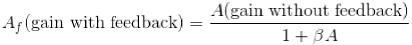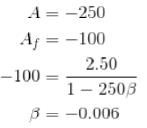Courses

# Bipolar Junction Transistor NAT Level -1

## 10 Questions MCQ Test Topic wise Tests for IIT JAM Physics | Bipolar Junction Transistor NAT Level -1

Description
This mock test of Bipolar Junction Transistor NAT Level -1 for Physics helps you for every Physics entrance exam. This contains 10 Multiple Choice Questions for Physics Bipolar Junction Transistor NAT Level -1 (mcq) to study with solutions a complete question bank. The solved questions answers in this Bipolar Junction Transistor NAT Level -1 quiz give you a good mix of easy questions and tough questions. Physics students definitely take this Bipolar Junction Transistor NAT Level -1 exercise for a better result in the exam. You can find other Bipolar Junction Transistor NAT Level -1 extra questions, long questions & short questions for Physics on EduRev as well by searching above.
*Answer can only contain numeric values
QUESTION: 1

### For a transistor circuit, VCE when Vin = 0V (in volts).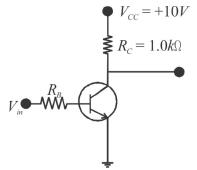Solution:

When Vin = 0V, the transistor is in cut-off (acts like an open switch) and VCE =VCC = 10V.

*Answer can only contain numeric values
QUESTION: 2

### For a BJT, the current amplification factor ∝ = 0.9 . This transistor is connected in common emitter configurations. When the base current changes by 0.4 mA, the change in collector current will be (in mA).

Solution: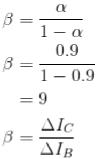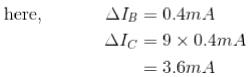*Answer can only contain numeric values
QUESTION: 3

### A power amplifier gives 150 W output of or an input of 1.5W. The gain in dB is :

Solution: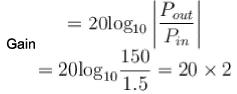= 40 dB

*Answer can only contain numeric values
QUESTION: 4

Calculate the collector voltage ( Vc ) in volts o f the transistor circuit as shown below.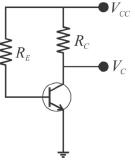Solution: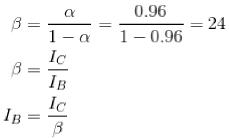By applying KVL,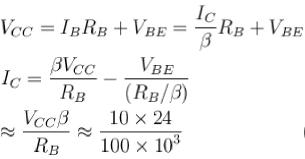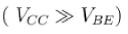lc = 2.4 mA
Now KVL in innerloop,VC= VCC- ICRC =10 - 2.4 x 10-3 x 2.2 x 103
VC = 4.72

*Answer can only contain numeric values
QUESTION: 5

Figure shows a common emitter amplifier with β = 100. What is the maximum peak to peak input signal (in mV) for which distortion free output may be obtained Assume VBE = 0 r= 20 Ω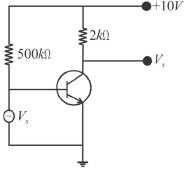Solution: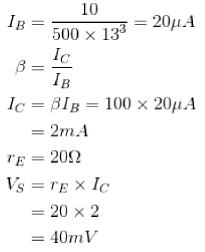But for maximum peak to peak input signal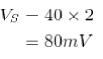*Answer can only contain numeric values
QUESTION: 6

In the following circuit, Tr1 and Tr2 are identical transistors having VBE = 0.7V. The current passing through the transistor Tr2 is (in mA).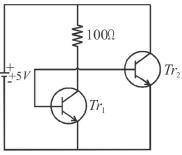Solution:

Applying KVL,
5=100x1 + 0.7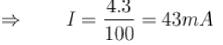*Answer can only contain numeric values
QUESTION: 7

If the transistor amplifier has a gain of 20dB, then the ratio of output to input power is :

Solution:

The ratio of output to input power is known as power amplification.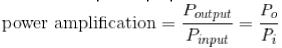The logarithm of the power amplification is represented in bel.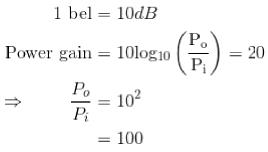*Answer can only contain numeric values
QUESTION: 8

A common emitter amplifier has voltage gain of 50, an input impedance of 1000Ω and an output impedance of 200Ω. The power gain of the amplifier will be (in dB).

Solution:

Power gain = Voltage gain x current gain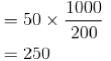*Answer can only contain numeric values
QUESTION: 9

The input signal given to a common emitter amplifier having a voltage gain of 20 is Vin = 20 cos (5t + 20°). The corresponding output signal has an amplitude equal to ?

Solution: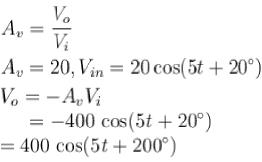*Answer can only contain numeric values
QUESTION: 10

The feedback ratio of an amplifier, which an amplification of a negative feedback, changes the voltage gain the voltage gain from - 250 to - 100 is (give magnitude only).

Solution: